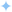Edit on GitHub

# useMutation Hook, Update Cache

let's create a ReScript module for create todo mutation in `src/components/Todo/TodoInput.res` with the following code

`module AddTodoMutation = %graphql(`  mutation(\$todo: String!, \$isPublic: Boolean!) {    insert_todos(objects: [{ title: \$todo, is_public: \$isPublic }]) {      affected_rows      returning {        id        title        created_at        is_completed      }    }  }`)`
```@react.componentlet make = (~isPublic=false) => {  let (todoInput, setTodoInput) = React.useState(_ => "")  let (mutate, _result) = AddTodoMutation.use()
let resetInput = () => {    setTodoInput(_ => "")  }
<form    className="formInput"    onSubmit={e => {      ReactEvent.Form.preventDefault(e)      mutate({todo: todoInput, isPublic: isPublic})      ->Js.Promise.then_(res => {        resetInput()        Js.Promise.resolve(res)      }, _)      ->ignore    }}>    <input      className="input"      value={todoInput}      placeholder="What needs to be done?"      onChange={e => setTodoInput(ReactEvent.Form.target(e)["value"])}    />    <i className="inputMarker fa fa-angle-right" />  </form>}```

Since `AddTodoMutation` is a GraphQL mutation query, `AddTodoMutation.use()` uses `useMutation` React hook of Apollo. In the `useMutation` React hook, the first argument of the result tuple is the mutate function; (addTodo) in this case. Read more about the mutate function here.

In the above component, mutate function is called with variables only. This will execute the mutation and change the data on server but it doesn't update Apollo cache. To update Apollo cache, we should also pass update option to mutate function in addition to variables as shown in the code below.

```@react.componentlet make = (~isPublic=false) => {  let (todoInput, setTodoInput) = React.useState(_ => "")  let (mutate, _result) = AddTodoMutation.use()
let resetInput = () => {    setTodoInput(_ => "")  }
<form    className="formInput"    onSubmit={e => {      ReactEvent.Form.preventDefault(e)      mutate(~update=({readQuery, writeQuery}, {data}) => {        if !isPublic {          switch data {          | Some({insert_todos}) =>            switch insert_todos {            | Some({returning}) =>              let newTodo: TodosQuery.t_todos = {                __typename: returning.__typename,                id: returning.id,                title: returning.title,                created_at: returning.created_at,                is_completed: returning.is_completed,              }              let existingTodosResponse = readQuery(~query=module(TodosQuery), ())              switch existingTodosResponse {              | Some(todosResult) =>                switch todosResult {                | Ok({todos}) => {                    let newTodos = Js.Array2.concat([newTodo], todos)                    let _ = writeQuery(~query=module(TodosQuery), ~data={todos: newTodos}, ())                  }                | _ => ()                }              | None => ()              }            | None => ()            }          | None => ()          }        } else {          ()        }      }, {todo: todoInput, isPublic: isPublic})->Js.Promise.then_(res => {        resetInput()        Js.Promise.resolve(res)      }, _)->ignore    }}>    <input      className="input"      value={todoInput}      placeholder="What needs to be done?"      onChange={e => setTodoInput(ReactEvent.Form.target(e)["value"])}    />    <i className="inputMarker fa fa-angle-right" />  </form>}```

ReScript enforces us to handle all possible cases with pattern matching. For example, data in the update method may or mayn't have insert_todos properties based on the query. So we have to handle both the cases with pattern matching as shown below

`switch data {  | Some({insert_todos}) => // logic to handle insert_todos  | None => // logic to handle None}`
•Build apps and APIs 10x faster
•Built-in authorization and caching
•8x more performant than hand-rolled APIs